# Gravity and Circular Motion RevisionPage 1

#### WATCH ALL SLIDES

Slide 1Gravity and Circular Motion Revision AQA syllabus A Section 13.3.1-6

B.W.Hughes

Slide 2## Circular motion

When an object undergoes circular motion it must experience a

centripetal force

This produces an acceleration

towards the centre of the circle

Slide 3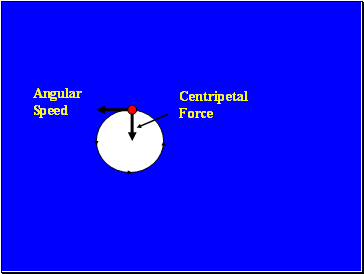Angular Speed

Centripetal Force

Slide 4Slide 5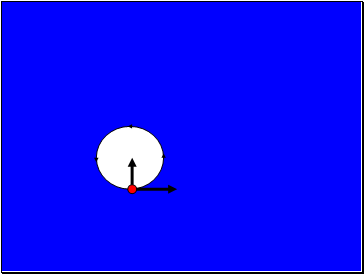Slide 6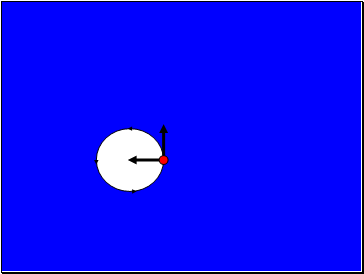Slide 7## Angular speed

Angular speed can be measured in ms-1 or

Revs-1 (revolutions per second)

The symbol for angular speed in radians per second is

ω

Slide 8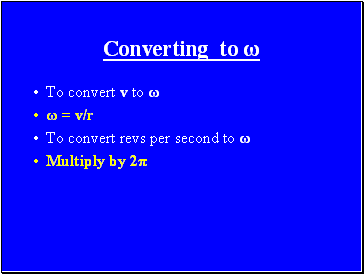## Converting to ω

To convert v to ω

ω = v/r

To convert revs per second to ω

Multiply by 2π

Slide 9Acceleration

The acceleration towards the centre of the circle is

a = v2/r OR

a = ω2r

Slide 10## Centripetal Force Equation

The general force equation is

F = ma

so the centripetal force equation is

F = mv2/r OR

F = m ω2r

THESE EQUATIONS MUST BE LEARNED!!

Slide 11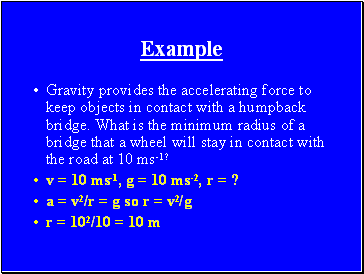## Example

Gravity provides the accelerating force to keep objects in contact with a humpback bridge. What is the minimum radius of a bridge that a wheel will stay in contact with the road at 10 ms-1?

v = 10 ms-1, g = 10 ms-2, r = ?

a = v2/r = g so r = v2/g

r = 102/10 = 10 m

Slide 12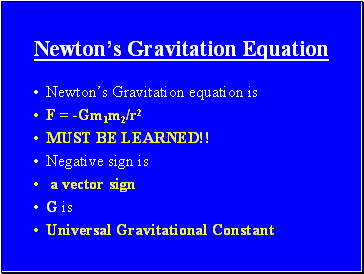## Newton’s Gravitation Equation

Newton’s Gravitation equation is

F = -Gm1m2/r2

MUST BE LEARNED!!

Negative sign is

a vector sign

G is

Universal Gravitational Constant

Slide 13m1 and m2 are

the two gravitating masses

r is

the distance between their centres of gravity

The equation is an example of an

Inverse square law

Slide 14

Go to page:
1  2  3